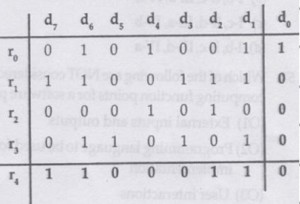Related Articles

# GATE | Gate IT 2008 | Question 64

• Last Updated : 09 Mar, 2018

Data transmitted on a link uses the following 2D parity scheme for error detection:
Each sequence of 28 bits is arranged in a 4×7 matrix (rows r0 through r3, and columns d7 through d1) and is padded with a column d0 and row r4 of parity bits computed using the Even parity scheme. Each bit of column d0 (respectively, row r4) gives the parity of the corresponding row (respectively, column). These 40 bits are transmitted over the data link.The table shows data received by a receiver and has n corrupted bits. What is the mini­mum possible value of n?
(A) 1
(B) 2
(C) 3
(D) 4

Explanation: In the given 2D parity matrix, all rows excepthave even parity. Therefore there must be atleast 1 bit error in this row.
Also, there are three columns with odd parities (odd parity indicates errors),,and.
So there must be a minimum of 3 bit errors.
All three errors could have occurred inor two of these errors could have occurred in any other row. Sincehas an odd parity, there is at least one bit-error in this row.
Therefore option (C) is correct.

This explanation is provided by Chirag Manwani.

Quiz of this Question

Attention reader! Don’t stop learning now.  Practice GATE exam well before the actual exam with the subject-wise and overall quizzes available in GATE Test Series Course.

Learn all GATE CS concepts with Free Live Classes on our youtube channel.

My Personal Notes arrow_drop_up